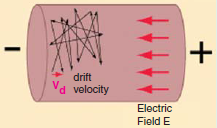# Drift Velocity of Electron

## Drift Velocity of Electron

To understand drift velocity, assume that there is a conductor.

And, there are n number of free electrons per m3 of the conductor.

Let us assume that the axial drift velocity of these electrons be v meters/second.

See the figure below to understand the situation.Now, we all know that Distance = Speed x Time .

Hence, In time dt, distance travelled by these electrons would be would be v x dt .

Now, let us assume A to be the area of cross-section of this conductor.

Then the volume would be vAdt

And the number of electrons contained in this volume would be nvAdt.

Obviously, all these electrons will cross the conductor cross-section in time dt.

If e is the charge of each electron, then total charge which crosses the section in time dt is dq=nAevdt.

Since current is the rate of flow of charge, it is given as

i = dq/dt

or,

i = nAev dt / dt

or,

i = nAev

Now, we know that Current density, J =i/A = nevA ampere/metre2

Assuming a normal current density J = 1.55 x 106 A/m2, n=1029 for a copper conductor and e = 1.6 x 1029 coulomb, we get the drift velocity to be about 0.58 cm/min. Thus we see that the electron drift velocity is very slow.

Now let us test our understanding of electron drift velocity with the help of an example.

Question : A conductor material has a free-electron density of 1024 electrons per metre3. When a voltage is applied, a constant drift velocity of 1.5×10-2 metre/second is attained by the electrons. If the cross-sectional area of the material is 1 cm2, calculate the magnitude of the current. Electronic charge is 1.6 x 10-19 coulomb.

Answer : The magnitude of the current is

i = nAev

Where,

n =1024

A= 1 cm2 = 10-4 m2

e = 1.6 x 10-19 C

v = 1.5 x 10-2 m/s

Substituting these values, we get :

i = 1024 x 10-4 x 1.6 x 10-19 x 1.5 x 10-2

or,

i = 0.24 A

Now, we understand the drift velocity of electron. However,  there are two more things that we need to understand:

• Velocity of charge
• Velocity of propagation of electric field

Velocity of charge is the speed with which charge drifts in a conductor.  It’s value is very low (fraction of a metre per second).

Velocity of propagation of electric field, is the speed with which the effect of e.m.f. is experienced at all parts of the conductor resulting in the flow of current. Its value is very high (3×108 m/s).